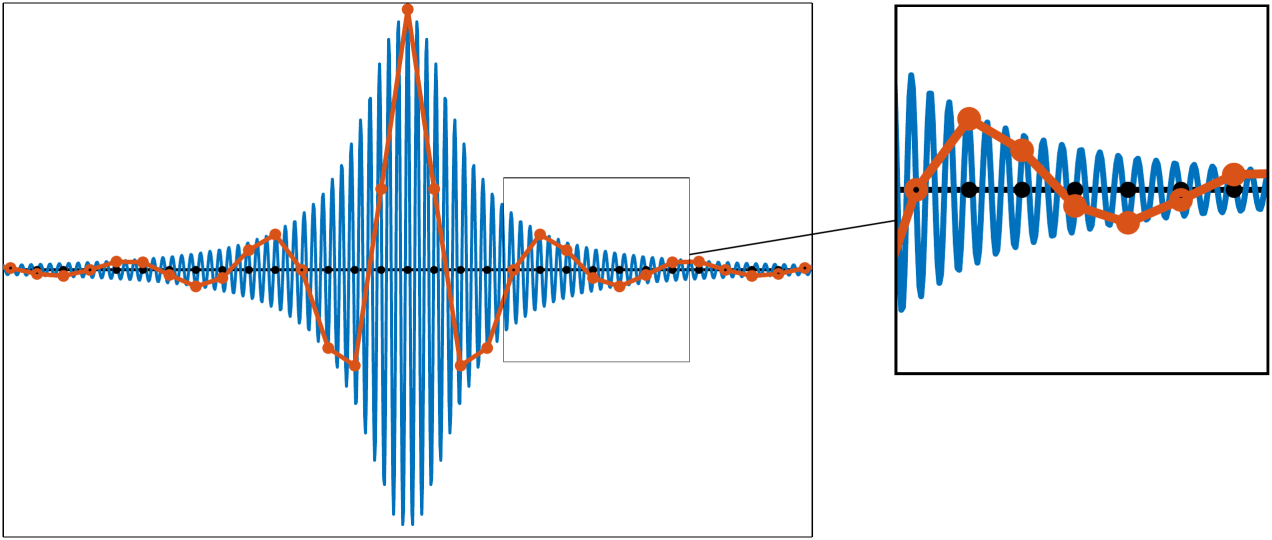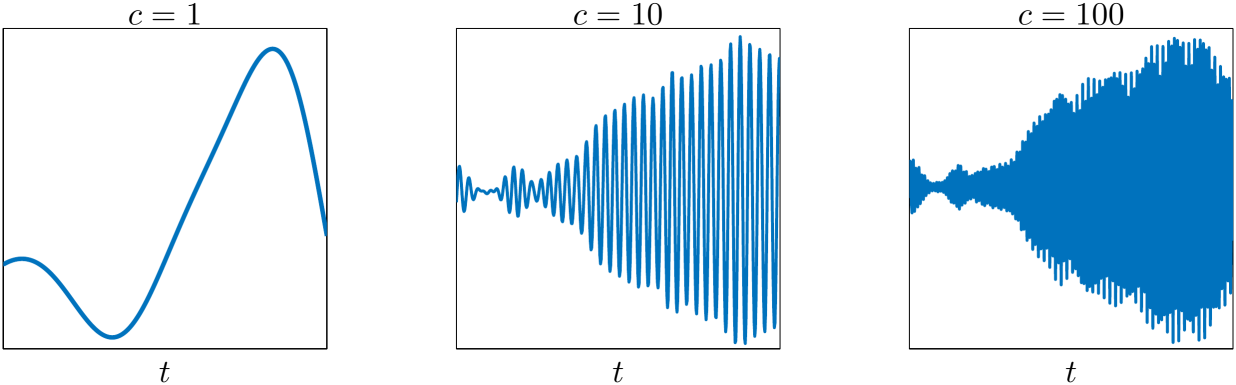# Project B1 • Klein-Gordon-Zakharov systems in high-frequency regimes (7/2015 - 6/2019)

### Principal investigators

 Prof. Dr. Guido Schneider (7/2015 - 6/2019) JProf. Dr. Katharina Schratz (7/2015 - 6/2019)

### Project summary

The aim of this project is to analyze the Klein-Gordon-Zakharov system in high plasma frequency and (simultaneous) subsonic regimes. Due to the highly oscillatory nature of the problem classical numerical schemes break down, in particular in certain singular limits. Therefore, we need to develop new analytic techniques and efficient numerical schemes to overcome severe step size restrictions and huge computational costs.

## Numerical challenges of highly oscillatory problems

If a solution of the underlying equation is highly oscillatory, it becomes very challenging for numerical methods to resolve the oscillations and to yield a good numerical approximation. In order to compute a numerical approximation of a highly oscillatory function (see Figure 1 below) we have to discretize the interval with a finite number of points. At these grid points we compute approximations of the function values.Figure 1 Plot of a highly oscillatory function in blue. Grid points of the discretization in black. Approximation in red.

Afterwards we construct our numerical solution by interpolating between the approximated values. The figure shows an example where the approximation fails completely even though we evaluate the function values exactly at the discrete (finite) points. We can improve the approximation by introducing a finer grid, which, however, requires much more computational and memory costs and therefore will not yield an efficient numerical scheme. This makes the efficient approximation of highly oscillatory differential equations an ongoing challenge in numerical analysis.

### The Klein-Gordon-Zakharov system

The starting point of this project was the Klein Gordon Zakhavov (KGZ) system $\begin{equation*} c^{-2}\partial_{tt}^2 z = \Delta z - c^2 z \pm nz, \\ \alpha^{-2}\partial_{tt}^2 n = \Delta n + \Delta \vert z\vert^2\quad\,\,\, \end{equation*}$ in certain high frequency regimes, i.e., for large $c$ and/or large $\alpha$, where the solution becomes highly oscillatory in time (see the Figure 2 below).Figure 2 Plot of the solution $z$ of the Klein-Gordon-Zakharov system for different values of $c$ at a fixed spatial point.

We are interested in a robust analytic and numerical description of the KGZ system for such values of $c$ and $\alpha$. Such a description can be obtained via the limit systems for $c\to\infty$ and/or $\alpha\to\infty$. This analysis is particularly of great interest from a numerical point of view: Correctly resolving this highly oscillatory behavior in the exact solution in these regimes is numerically very delicate.

Severe time step restrictions need to be imposed and this requires a great deal of computational costs. An asymptotic ansatz allows to construct efficient time integrators as the highly oscillatory parts in the exact solution could be filtered out explicitly. Thus, the numerical task could be reduced to the time integration of the corresponding non-oscillatory limit systems, which can be carried out very efficiently without any additional time step restriction.

The construction of such robust, efficient time integrators involves a careful analysis of the numerical time integrators for the corresponding limit systems and rigorous error estimates between true solutions of the original system and the approximations constructed via the limit system.

The KGZ system turned out to be a well chosen prototype problem since the tools developed for the KGZ system can be used to establish similar results for a wider class of problems. Moreover, extending the original question, uniformly accurate methods for KGZ like systems have been developed, i.e., the numerical costs for such schemes are independent of the $c$ and $\alpha$ and not only valid for $c$ large and/or $\alpha$ large.

• Analytic results that the various limit systems make correct predictions about the dynamics for $c$ large and/or $\alpha$ large can be found in [DSS16] and [BSSZ19].
In the first paper it turned out that limit systems in the limit $c$ fixed and $\alpha\to\infty$ depends whether the KGZ system is posed on $\mathbb{R}^3$ or $\mathbb{T}^3$. The abstract approximation theorem in the second paper applies to a number of semilinear systems, such as the Dirac-Klein-Gordon system, the Klein-Gordon-Zakharov system, and a mean field polaron model. It extracts the common features of scattered results in the literature, but also gains an approximation result for the Dirac-Klein-Gordon system which has not been documented in the literature before. The abstract approximation theorem is sharp in the sense that there exists a quasilinear system of the same structure, namely the Zakharov system with a 'wrong' sign in the nonlinearity, where the regular limit system, namely the NLS equation, makes wrong predictions.

• We propose asymptotic consistent exponential-type integrators for the Klein-Gordon-Schrödinger system. This novel class of integrators allows us to solve the system from slowly varying relativistic up to challenging highly oscillatory non-relativistic regimes without any step size restriction. In particular, our first- and second-order exponential-type integrators are asymptotically consistent in the sense of asymptotically converging to the corresponding decoupled free Schrödinger limit system. See [BKS18].

• We introduce efficient and robust exponential-type integrators for Klein-Gordon equations which resolve the solution in the relativistic regime as well as in the highly-oscillatory nonrelativistic regime without any step-size restriction under the same regularity assumptions on the initial data required for the integration of the corresponding nonlinear Schrödinger (NLS) limit system. In contrast to previous works we do not employ any asymptotic/multiscale expansion of the solution. This allows us to derive uniform convergent schemes under far weaker regularity assumptions on the exact solution. In addition, the newly derived first- and second-order exponential-type integrators converge to the classical Lie, respectively, Strang splitting in the nonlinear Schrödinger limit. See [BFS18].

• We present a novel class of oscillatory integrators for the KGZ system which are uniformly accurate with respect to the plasma frequency $c$. Convergence holds from the slowly-varying low-plasma up to the highly-oscillatory high-plasma frequency regimes without any step size restriction and, especially, uniformly in $c$. The introduced scheme is moreover asymptotic consistent and approximates the solutions of the corresponding Zakharov limit system in the high-plasma frequency limit $c\to\infty$. We establish rigorous error estimates for the the introduced oscillatory integrator and numerically underline its uniform convergence property. The derivation of uniformly accurate methods for the KGZ system in the subsonic limit regime ($\alpha\to\infty$) and also in the simultaneous limit regimes ($(c,\alpha)\to\infty$) will be the subject of future research. See [BS19].

## Publications

1. , , and . Effective numerical simulation of the Klein–Gordon–Zakharov system in the Zakharov limit. In W. Dörfler, M. Hochbruck, D. Hundertmark, W. Reichel, A. Rieder, R. Schnaubelt, and B. Schörkhuber, editors, Mathematics of Wave Phenomena, Trends in Mathematics, pages 37–48, October . Birkhäuser Basel. [bibtex]

2. and . Asymptotic preserving trigonometric integrators for the quantum Zakharov system. BIT, 1–21, June . Online first. [preprint] [bibtex]

3. , , and . Randomized exponential integrators for modulated nonlinear Schrödinger equations. IMA J. Numer. Anal., 1–20, January . Online first. [preprint] [bibtex]

4. , , and . Splitting methods for nonlinear Dirac equations with Thirring type interaction in the nonrelativistic limit regime. J. Computat. Appl. Math., 112494, September . Online first, in press. [preprint] [bibtex]

5. , , , and . Effective slow dynamics models for a class of dispersive systems. J. Dyn. Diff. Equat., 1–33, September . Online first. [preprint] [bibtex]

6. , , , , and . Trigonometric integrators for quasilinear wave equations. Math. Comp., 88(316):717–749, March . [preprint] [bibtex]

7. and . Uniformly accurate oscillatory integrators for the Klein–Gordon–Zakharov system from low- to high-plasma frequency regimes. SIAM J. Numer. Anal., 57(1):429–457, February . [preprint] [bibtex]

8. and . Low regularity exponential-type integrators for semilinear Schrödinger equations. Found. Comput. Math., 18(3):731–755, June . [preprint] [bibtex]

9. , , and . Uniformly accurate exponential-type integrators for Klein–Gordon equations with asymptotic convergence to the classical NLS splitting. Math. Comp., 87(311):1227–1254, May . [preprint] [bibtex]

10. , , and . Asymptotic consistent exponential-type integrators for Klein–Gordon–Schrödinger systems from relativistic to non-relativistic regimes. Electron. Trans. Numer. Anal., 48:63–80, March . [bibtex]

11. and . Trigonometric time integrators for the Zakharov system. IMA J. Numer. Anal., 37(4):2042–2066, October . [preprint] [bibtex]

12. and . An exponential-type integrator for the KdV equation. Numer. Math., 136(4):1117–1137, August . [preprint] [bibtex]

13. and . Efficient time integration of the Maxwell–Klein–Gordon equation in the non-relativistic limit regime. J. Comput. Appl. Math., 316:247–259, May . Selected Papers from NUMDIFF-14. [preprint] [bibtex]

14. , , and . From the Klein–Gordon–Zakharov system to the Klein–Gordon equation. Math. Methods Appl. Sci., 39(18):5371–5380, December . [preprint] [bibtex]

## Preprints

1. and . Asymptotic preserving trigonometric integrators for the quantum Zakharov system. CRC 1173 Preprint 2019/4, Karlsruhe Institute of Technology, January . [bibtex]

2. and . On the comparison of asymptotic expansion techniques for the nonlinear Klein–Gordon equation in the nonrelativistic limit regime. CRC 1173 Preprint 2018/52, Karlsruhe Institute of Technology, December . [bibtex]

3. , , and . Randomized exponential integrators for modulated nonlinear Schrödinger equations. CRC 1173 Preprint 2018/49, Karlsruhe Institute of Technology, December . [bibtex]

4. . The KdV approximation for a system with unstable resonances. CRC 1173 Preprint 2018/42, Karlsruhe Institute of Technology, November . [bibtex]

## Theses

1. . Uniformly accurate methods for Klein–Gordon type equations. PhD thesis, Karlsruhe Institute of Technology (KIT), July . [bibtex]

2. . Numerical integrators for Maxwell–Klein–Gordon and Maxwell–Dirac systems in highly to slowly oscillatory regimes. PhD thesis, Karlsruhe Institute of Technology (KIT), August . [bibtex]

Project-specific staff
Name Phone E-Mail
Former staff
Name Title Function
Dr. Doctoral Researcher
Dr. Doctoral Researcher
Prof. Dr. Principal investigator
JProf. Dr. Member & scientific researcher